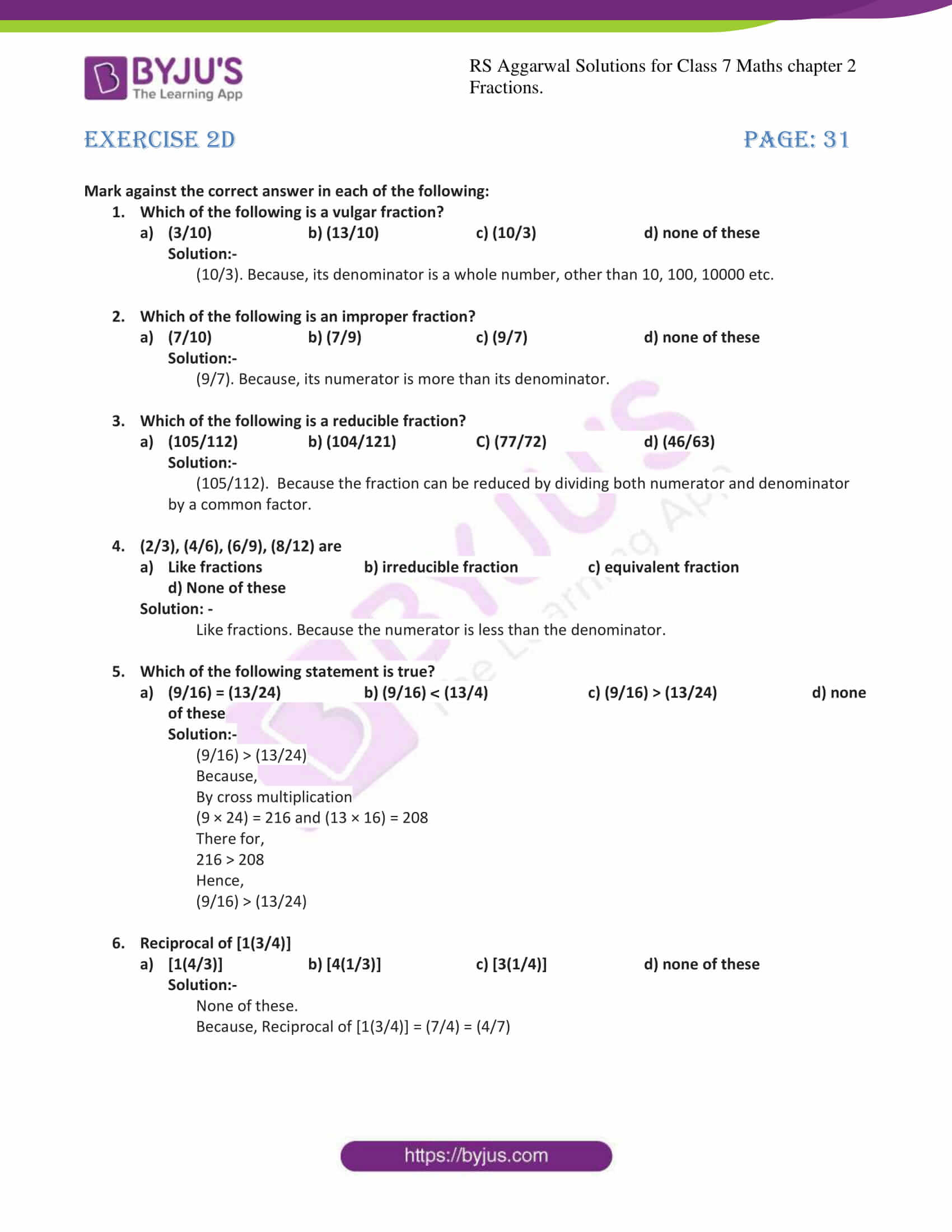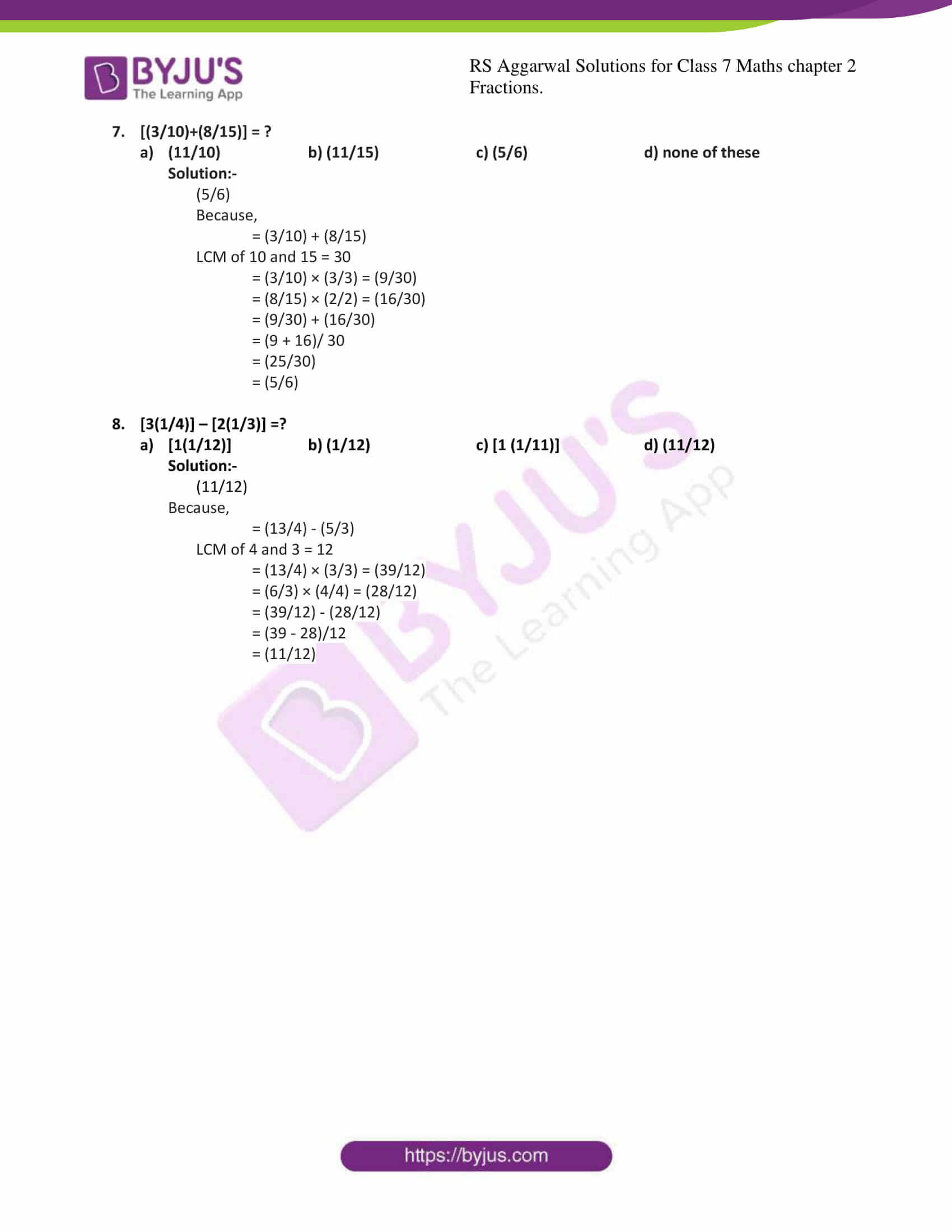# RS Aggarwal Solutions For Class 7 Maths Exercise 2D Chapter 2 Fractions

The pdf of RS Aggarwal Solutions for the Exercise 2D of Class 7 Maths Chapter 2, Fractions is the best study material for those students who are finding complications in solving problems. These solutions can help students clear doubts quickly and assist in learning the topic effectively. Our expert teachers formulate these exercises to help you with your exam preparation to achieve good marks in Maths. Students who wish to score good marks in Maths practise RS Aggarwal Class 7 Solutions.

## Download the PDF of RS Aggarwal Solutions For Class 7 Maths Chapter 2 Fractions – Exercise 2D### Access answers to Maths RS Aggarwal Solutions for Class 7 Chapter 2 – Fractions Exercise 2D

Mark against the correct answer in each of the following:

1. Which of the following is a vulgar fraction?
a) (3/10) b)(13/10) c)(10/3) d)none of these

Solution:-

(10/3). Because, its denominator is a whole number, other than 10, 100, 10000 etc.

2. Which of the following is an improper fraction?
a)(7/10) b)(7/9) c)(9/7) d)none of these

Solution:-

(9/7). Because, its numerator is more than its denominator.

3. Which of the following is a reducible fraction?
a) (105/112) b)(104/121) C)(77/72) d)(46/63)

Solution:-

(105/112). Because the fraction can be reduced by dividing both numerator and denominator by a common factor.

4. (2/3), (4/6), (6/9), (8/12) are
a)Like fractions b)irreducible fraction c)equivalent fraction

d) None of these

Solution: –

Like fractions. Because the numerator is less than the denominator.

5. Which of the following statement is true?
a)(9/16) = (13/24) b)(9/16) < (13/4) c)(9/16) > (13/24) d)none of these

Solution:-

(9/16) > (13/24)

Because,

By cross multiplication

(9 × 24) = 216 and (13 × 16) = 208

There for,

216 > 208

Hence,

(9/16) > (13/24)

6. Reciprocal of [1(3/4)]
a)[1(4/3)] b)[4(1/3)] c)[3(1/4)] d)none of these

Solution:-

None of these.

Because, Reciprocal of [1(3/4)] = (7/4) = (4/7)

7. [(3/10)+(8/15)] = ?
a)(11/10) b)(11/15) c)(5/6) d)none of these

Solution:-

(5/6)

Because,

= (3/10) + (8/15)

LCM of 10 and 15 = 30

= (3/10) × (3/3) = (9/30)

= (8/15) × (2/2) = (16/30)

= (9/30) + (16/30)

= (9 + 16)/ 30

= (25/30)

= (5/6)

8. [3(1/4)] – [2(1/3)] =?
a)[1(1/12)] b)(1/12) c)[1 (1/11)] d)(11/12)

Solution:-

(11/12)

Because,

= (13/4) – (5/3)

LCM of 4 and 3 = 12

= (13/4) × (3/3) = (39/12)

= (6/3) × (4/4) = (28/12)

= (39/12) – (28/12)

= (39 – 28)/12

= (11/12)

### RS Aggarwal Solutions for Class 7 Maths Exercise 2D Chapter – 2 Fractions

RS Aggarwal Solutions For Class 7 Maths Chapter 2 Fractions Exercise 2D has objective type questions. In this exercise, application-level questions are covered to make the students think in various ways to get the answer. The objective type questions in this exercise are based on all the topics covered in this chapter. Students are advised to try solving the problems from RS Aggarwal Solutions book of Class 7.